# TensorFlow实现CNN卷积神经网络对手写数字集mnist的模型训练

mnist手写数字集相当于是TensorFlow应用中的Helloworld。

## 一、mnist手写数字数据集

MNIST是深度学习的经典入门demo，他是由6万张训练图片和1万张测试图片构成的，每张图片都是28*28大小（如下图），而且都是黑白色构成（这里的黑色是一个0-1的浮点数，黑色越深表示数值越靠近1），这些图片是采集的不同的人手写从0到9的数字。TensorFlow将这个数据集和相关操作封装到了库中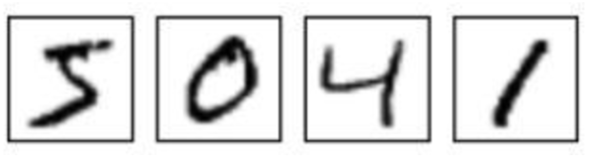我们可以访问官方网站进行数据下载，当然也可以通过TensorFlow进行调用：

http://yann.lecun.com/exdb/mnist/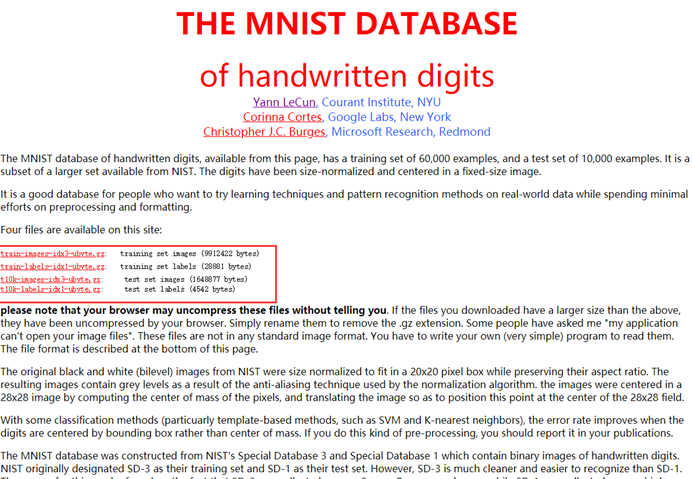注意下载的时候要将4个红色的包全部下载好。

## 二、我们要构建一个什么样的卷积神经网络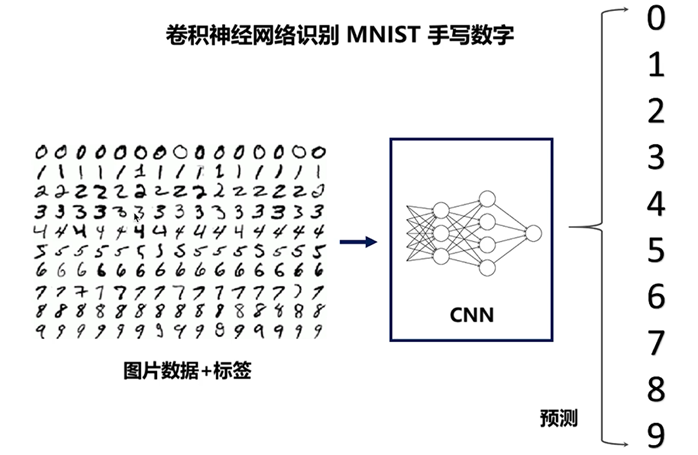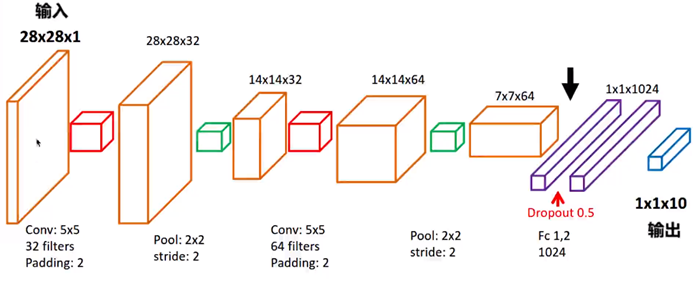上面的这张图，就是我们将要构建的卷积神经网络，我们的构建步骤是：

•         输入28*28*1的数据集
•         第一个红色方块，代表第1层卷积，下面是它的相关参数，形状变为[28, 28, 32]；
•         第一个绿色方块，代表第1层池化，或者是亚采样，下方是它的相关参数，形状变为[14, 14, 32]；
•         第二个红色方块，代表第2层卷积，下面是它的相关参数，形状变为[14, 14, 64]；
•         第二个绿色方块，代表第2层池化，或者是亚采样，下方是它的相关参数，形状变为[7, 7, 64]；
•         进行平坦化，形成1024神经元的全连接层，形状为[1, 1, 1024]；
•         丢弃50%，即使rate=0.5；
•         形成10个神经元的全连接层，输出形状[1, 1, 10]

这就是我们构建的一个简单的卷积神经网络。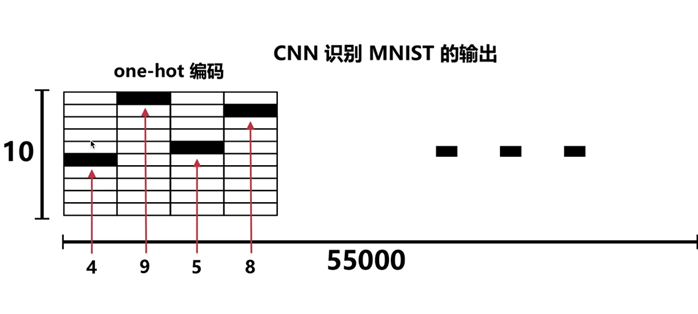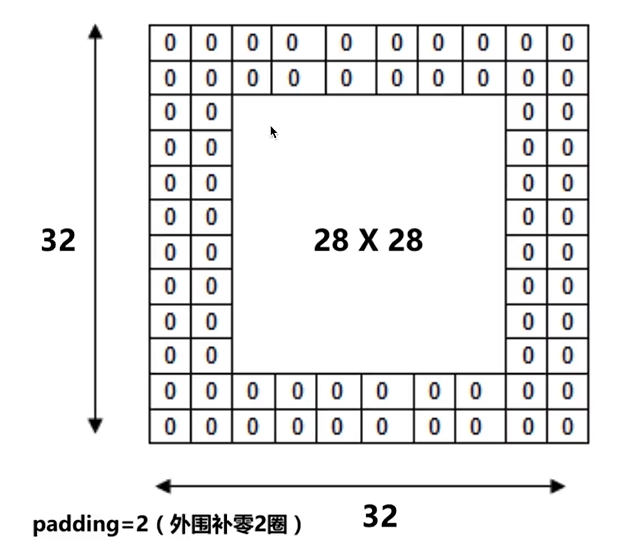## 三、如何使用TensorFlow进行构建

### 1.导入相关库，通过网络下载并接收手写mnist数字库

import numpy as np
import tensorflow as tf
# 下载并载入MNIST手写数字库(55000 * 28 * 28)55000张训练图像
import tensorflow.examples.tutorials.mnist.input_data as input_data

# 接收手写数据
mnist = input_data.read_data_sets('Mnist_Data/', one_hot=True)

其中，one_hot是一种编码形式，独热码的编码（encoding）形式

0， 1， 2， 3， 4， 5， 6， 7， 8， 9的十位数字

one_hot=True 就会表示成下面的编码模式：

# 0:1000000000
# 1:0100000000
# 2:0010000000
# 3:0001000000
# 4:0000100000
# 5:0000010000
# 6:0000001000
# 7:0000000100
# 8:0000000010
# 9:0000000001

### 2.设置卷积神经网络输入张量及测试数据

# None 表示张量（Tensor）的第一个维度可以是任何长度
input_x = tf.placeholder(tf.float32, [None, 28*28]) / 255.
output_y = tf.placeholder(tf.int32, [None, 10]) # 输出：10个数字的标签
input_x_images = tf.reshape(input_x, [-1, 28, 28, 1]) # 改变形状之后的输入

# Test（测试）数据集里选取3000个手写数字的图片和对应标签
test_x = mnist.test.images[:3000] # 图片
test_y = mnist.test.labels[:3000] # 标签

None 表示张量（Tensor）的第一个维度可以是任何长度。

Test（测试）数据集里选取3000个手写数字的图片和对应标签。

### 3.分层构建卷积神经网络

# 第1层卷积
conv1 = tf.layers.conv2d(
inputs=input_x_images,	# 形状 [28， 28， 1]
filters=32,		# 32个过滤器， 输出的深度（depth）是32
kernel_size=[5, 5],	# 过滤器在二维的大小是(5 * 5)
strides=1,		# 步长是1
activation=tf.nn.relu	# 激活函数是Relu
)	# 形状 [28, 28, 32]

# 第1层池化（亚采样）
pool1 = tf.layers.max_pooling2d(
inputs=conv1,			# 形状 [28, 28, 32]
pool_size=[2, 2],		# 过滤器在二维的大小是(2 * 2)
strides=2			# 步长是2
)	# 形状[14, 14, 32]

# 第2层卷积
conv2 = tf.layers.conv2d(
inputs=pool1,			# 形状 [14, 14, 32]
filters=64,			# 64个过滤器， 输出的深度（depth）是64
kernel_size=[5, 5],		# 过滤器在二维的大小是(5 * 5)
strides=1,			# 步长是1
activation=tf.nn.relu		# 激活函数是Relu
)	# 形状 [14, 14, 64]

# 第2层池化（亚采样）
pool2 = tf.layers.max_pooling2d(
inputs=conv2,			# 形状 [14, 14, 64]
pool_size=[2, 2]	,	# 过滤器在二维的大小是(2 * 2)
strides=2			# 步长是2
)	# 形状[7, 7, 64]

# 平坦化（flat）
flat = tf.reshape(pool2, [-1, 7 * 7 *64])	# 形状 [7 * 7 * 64]

# 1024 个神经元的全连接层
dense = tf.layers.dense(inputs=flat, units=1024, activation=tf.nn.relu)

# Dropout：丢弃50%，rate=0.5
dropout = tf.layers.dropout(inputs=dense, rate=0.5)

# 10个神经元的全连接层，这里不用激活函数做非线性化
logits = tf.layers.dense(inputs=dropout, units=10)	# 输出形状[1, 1, 10]

上面的代码，按照我们在第二部门中阐述的神经网络进行了构建，

其中tf.layers.conv2d；tf.layer.max_poling2d；tf.layer.dense；tf.layers.dropout等都会涉及到很多的参数，这些在TensorFlow官网的API文档中都有介绍，进行合理设置即可。

经过一系列地处理，最终输出了[1, 1, 10]的形状。

### 4.最小化误差及计算精度

# 计算误差（计算Cross entropy（交叉熵），再用Softmax计算出百分比概率）
loss = tf.losses.softmax_cross_entropy(onehot_labels=output_y, logits=logits)

# 计算预测值和实际标签的匹配程度（精度）
# 返回（accuracy, update_op）,会创建两个局部变量
accuracy = tf.metrics.accuracy(
labels=tf.argmax(output_y, axis=1),
predictions=tf.argmax(logits, axis=1),
)

### 5.训练神经网络并测试

# 创建会话
sess = tf.Session()

# 初始化变量：
init = tf.group(tf.global_variables_initializer(), tf.local_variables_initializer())
sess.run(init)

# 训练神经网络
for i in range(20000):
batch = mnist.train.next_batch(50) #从Train（训练)数据集里取下一个50样本
train_loss, _ = sess.run([loss, train_op], {input_x: batch, output_y: batch})
if i % 100 == 0:
test_accuracy = sess.run(accuracy, {input_x: test_x, output_y: test_y})
print("Step=%d, Train loss=%.4f, [Test accuracy=%.2f]" % (i, train_loss, test_accuracy))

# 测试：打印20个测试值和真实值
test_output = sess.run(logits, {input_x: test_x[:20]})
inferenced_y = np.argmax(test_output, 1)
print(inferenced_y, 'Inferenced numbers') # 推测的数字
print(np.argmax(test_y[:20], 1), 'Real numbers') # 真实的数字

# 关闭会话
sess.close()

通过创建session会话，进行神经网络的训练，为了得到一个较好的模型，我们训练20000步，最后使用20个数据进行测试。由于我使用的是Ubuntu虚拟机，内容只设置了2GB，所以20000步的训练会消耗1-2小时的时间。

## 四、程序运行结果

### 1.训练过程打印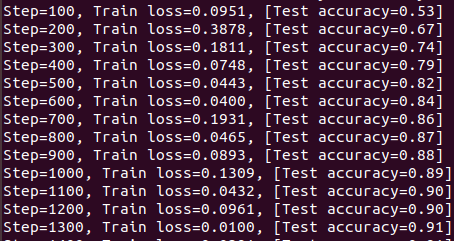在训练开始的时候，损失量不稳定，但是准确度提升很快，迅速达到了90%。

但随着训练的进行，准确率的提升逐渐变慢，当到达10000步左右的时候，准确率就达到98% 。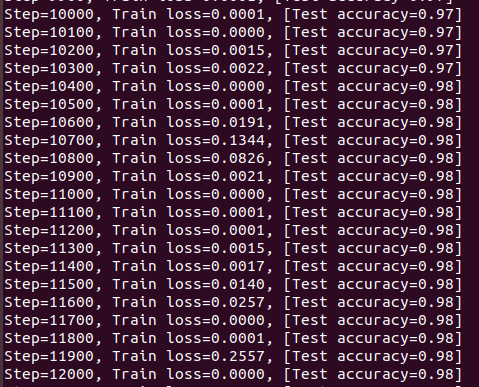在20000步训练完成的时候，准确率最后定格到了98%。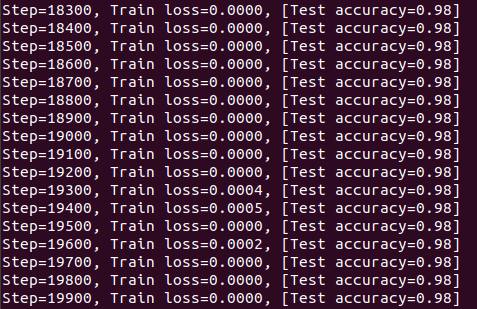### 2.测试数据

可以看到最后程序非常完美地完成了测试：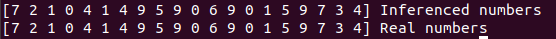可以看到上面的一行数组标签是预测的数字，下面的一行是实际的数据，正确率是100%，怎么样，赶紧开始试一下吧！

## 五、附完整代码加注释

# -*- coding: UTF-8 -*-

import numpy as np
import tensorflow as tf
# 下载并载入MNIST手写数字库(55000 * 28 * 28)55000张训练图像
import tensorflow.examples.tutorials.mnist.input_data as input_data

# 接收手写数据
mnist = input_data.read_data_sets('Mnist_Data/', one_hot=True)

# one_hot 是一种编码形式，独热码的编码（encoding）形式
# one_hot=True 就会表示成下面的编码模式
# 0， 1， 2， 3， 4， 5， 6， 7， 8， 9的十位数字
# 0:1000000000
# 1:0100000000
# 2:0010000000
# 3:0001000000
# 4:0000100000
# 5:0000010000
# 6:0000001000
# 7:0000000100
# 8:0000000010
# 9:0000000001

# None 表示张量（Tensor）的第一个维度可以是任何长度
input_x = tf.placeholder(tf.float32, [None, 28*28]) / 255.
output_y = tf.placeholder(tf.int32, [None, 10]) # 输出：10个数字的标签
input_x_images = tf.reshape(input_x, [-1, 28, 28, 1]) # 改变形状之后的输入

# Test（测试）数据集里选取3000个手写数字的图片和对应标签
test_x = mnist.test.images[:3000] # 图片
test_y = mnist.test.labels[:3000] # 标签

# 构建我们的卷积神经网络
# 第1层卷积
conv1 = tf.layers.conv2d(
inputs=input_x_images,	# 形状 [28， 28， 1]
filters=32,		# 32个过滤器， 输出的深度（depth）是32
kernel_size=[5, 5],	# 过滤器在二维的大小是(5 * 5)
strides=1,		# 步长是1
activation=tf.nn.relu	# 激活函数是Relu
)	# 形状 [28, 28, 32]

# 第1层池化（亚采样）
pool1 = tf.layers.max_pooling2d(
inputs=conv1,			# 形状 [28, 28, 32]
pool_size=[2, 2],		# 过滤器在二维的大小是(2 * 2)
strides=2			# 步长是2
)	# 形状[14, 14, 32]

# 第2层卷积
conv2 = tf.layers.conv2d(
inputs=pool1,			# 形状 [14, 14, 32]
filters=64,			# 64个过滤器， 输出的深度（depth）是64
kernel_size=[5, 5],		# 过滤器在二维的大小是(5 * 5)
strides=1,			# 步长是1
activation=tf.nn.relu		# 激活函数是Relu
)	# 形状 [14, 14, 64]

# 第2层池化（亚采样）
pool2 = tf.layers.max_pooling2d(
inputs=conv2,			# 形状 [14, 14, 64]
pool_size=[2, 2]	,	# 过滤器在二维的大小是(2 * 2)
strides=2			# 步长是2
)	# 形状[7, 7, 64]

# 平坦化（flat）
flat = tf.reshape(pool2, [-1, 7 * 7 *64])	# 形状 [7 * 7 * 64]

# 1024 个神经元的全连接层
dense = tf.layers.dense(inputs=flat, units=1024, activation=tf.nn.relu)

# Dropout：丢弃50%，rate=0.5
dropout = tf.layers.dropout(inputs=dense, rate=0.5)

# 10个神经元的全连接层，这里不用激活函数做非线性化
logits = tf.layers.dense(inputs=dropout, units=10)	# 输出形状[1, 1, 10]

# 计算误差（计算Cross entropy（交叉熵），再用Softmax计算出百分比概率）
loss = tf.losses.softmax_cross_entropy(onehot_labels=output_y, logits=logits)

# 计算预测值和实际标签的匹配程度（精度）
# 返回（accuracy, update_op）,会创建两个局部变量
accuracy = tf.metrics.accuracy(
labels=tf.argmax(output_y, axis=1),
predictions=tf.argmax(logits, axis=1),
)

# 创建会话
sess = tf.Session()

# 初始化变量：
init = tf.group(tf.global_variables_initializer(), tf.local_variables_initializer())
sess.run(init)

# 训练神经网络
for i in range(20000):
batch = mnist.train.next_batch(50) #从Train（训练)数据集里取下一个50样本
train_loss, _ = sess.run([loss, train_op], {input_x: batch, output_y: batch})
if i % 100 == 0:
test_accuracy = sess.run(accuracy, {input_x: test_x, output_y: test_y})
print("Step=%d, Train loss=%.4f, [Test accuracy=%.2f]" % (i, train_loss, test_accuracy))

# 测试：打印20个测试值和真实值
test_output = sess.run(logits, {input_x: test_x[:20]})
inferenced_y = np.argmax(test_output, 1)
print(inferenced_y, 'Inferenced numbers') # 推测的数字
print(np.argmax(test_y[:20], 1), 'Real numbers') # 真实的数字

# 关闭会话
sess.close()